• 六自由度机械臂研究（3）- 机械臂DH参数表建立 DH参数简介 DH参数全称是Denavit-Hartenberg参数， 目前是机器人领域广泛应用于机器人结构描述的方法。 我们可以用这些参数来来描述一个机器人机构， 并用这些参数来...
六自由度机械臂研究（3）- 机械臂DH参数表的建立
DH参数简介
DH参数全称是Denavit-Hartenberg参数， 目前是机器人领域广泛应用于机器人结构描述的方法。 我们可以用这些参数来描述一个机器人机构， 并用这些参数来进行运动学算法的推导与解算。 例如，对于一个六关节机械臂， 仅仅需要18个参数就可以完全描述这些固定的运动学参数。
因此， 机器人的每个连杆的可以用4个运动学参数来描述， 其中两个参数用于描述连杆本身， 另外两个参数用于描述连杆之间的连接关系。简单的说， 就是用这四个参数来描述两个相邻关节杆的相对位置关系。
关于连杆连接的两种描述： 标准DH建模， 改进DH建模， 根据我们的建系方法， 我们需要用改进DH建模法来进行建模， 两种建模的区别网上有很多讲解， 博主在此不多做详解， 博主参考博客为标准DH建模与改进DH建模 。
DH选的四个参数的物理意义
DH选的四个参数都有非常明确的物理含义：
link length （连杆长度）：两个关节的轴（旋转关节的旋转轴，平移关节的平移轴）之间的公共法线长度。
link twist  (连杆扭转 ）：一个关节的轴相对于另一个关节的轴绕它们的公共法线旋转的角度。
link offset  （连杆偏移）：一个关节与下一个关节的公共法线和它与上一个关节的公共法线沿这个关节轴的距离。
joint angle （关节转角）：一个关节与下一个关节的公共法线和它与上一个关节的公共法线绕这个关节轴的转角。
参数具体计算方法：
连杆长度 ai-1 ： 沿着Xi-1轴， 从Zi-1轴到Zi轴的距离。（表示连杆i-1两端关节轴的公垂线长度）
连杆扭转 αi-1 ：  绕着Xi-1轴， 从Zi-1轴到Zi轴的角度。
连杆偏距  di ： 沿Zi轴， 从 Xi-1轴到Xi轴的距离。
关节转角   θi： 绕Zi轴， 从Xi-1轴到Xi轴的角度。
根据已经建立好的六自由度机械臂的坐标系。 如下图所示：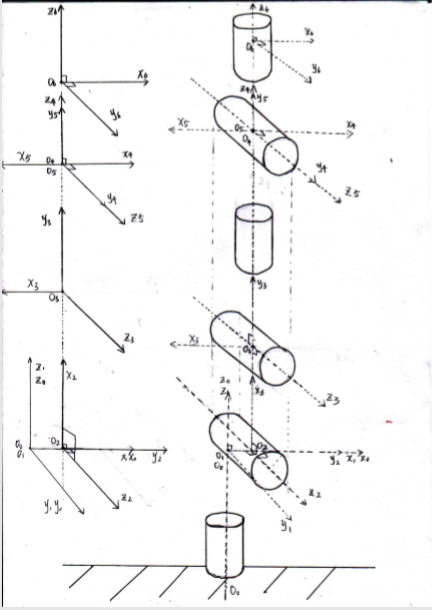我们可以根据以上方法来分别求出从坐标系{ 0 } 到  坐标系{5} 的四个DH参数。
根据改装过的六自由度机械臂的坐标系， 并通过上述DH参数计算方法（以当前姿势作为初始状态）， 可以得到DH参数表. 如下图所示：

i
ai-1 (cm)
αi-1
di (cm)
θi

1
0.00
0
0
0

2
1.25
pi/2
0
pi/2

3
10.5
0
0
pi/2

4
0.00
pi/2
13.0
-pi

5
0.00
pi/2
0
pi

6
0.00
pi/2
10.0
-pi

检查确认无误后， 就可以以DH参数表为基础建立各个关节轴的位姿方位矩阵。 这些矩阵就是运动学算法的基础元素。 下一节将会详细介绍。


展开全文教程
•matlab
• ## 机械臂DH参数总结

千次阅读 2020-09-15 15:22:42
DH参数（Denavit–Hartenberg parameters）是一个用四个参数表达两对关节连杆之间位置角度关系的机械臂数学模型和坐标系确定系统。 DH选的四个参数都的含义如下： link length（连杆长度）：两个关节的轴（旋转关节...
DH参数
DH参数（Denavit–Hartenberg parameters）是一个用四个参数表达两对关节连杆之间位置角度关系的机械臂数学模型和坐标系确定系统。DH选的四个参数都的含义如下：
link length（连杆长度）：两个关节的轴（旋转关节的旋转轴，平移关节的平移轴）之间的公共法线长度
link twist（连杆扭转）：一个关节的轴相对于另一个关节的轴绕它们的公共法线旋转的角度
link offset（连杆偏移）：一个关节与下一个关节的公共法线和它与上一个关节的公共法线沿这个关节轴的距离
joint angle（关节转角）：一个关节与下一个关节的公共法线和它与上一个关节的公共法线绕这个关节轴的转角
在DH参数体系里，把一个关节的轴（axis）定为z轴；公共法线（common normal）定为x轴，且x轴的方向为从本关节指向下一个关节。
连杆长度a，连杆偏移d，连杆扭转α，关节转角θ，也可表示如下图:展开全文• 一个串联机械臂是由多个连杆和运动副组成的运动链。通过运动副的连接，一个连杆能相对于另一个连杆做相对运动。运动链的一端，即机械手的基座，通常上是固定不动的，而运动链另一端上一般有夹持工具或者末端执行器，...
运动学是力学的一个分支，它在不考虑质量和作用力的前提下研究一个刚体，或者由多个刚体组成系统的运动。一个串联机械臂是由多个连杆和运动副组成的运动链。通过运动副的连接，一个连杆能相对于另一个连杆做相对运动。运动链的一端，即机械手的基座，通常上是固定不动的，而运动链另一端上一般有夹持工具或者末端执行器，可以在空间中自由运动。
一个串联机械臂的整个链路是由一组被称为连杆的刚体组成的，这些连杆由关节（运动副）连接起来。每个关节有一个平移（滑动或移动副）或者转动（转动副）的自由度。关节的运动改变了其邻接连杆的相对角度和位置。通常大部分机器人的关节都是回转的。
一个机器人的关节结构可以用一串字符来描述，例如 Puma560 为“RRRRRR”，斯坦福臂为“RRPRRR”，其中每个字符代表相应关节的类型，R是转动副，P为移动副。迪拉维特（Denavit）和哈滕贝格（Hartenberg）于1955年提出了一种用于描述这种串联式链路上连杆和关节几何关系的系统方法，这就是如今熟知的 D-H 参数法。
有 $N$ 个关节（编号从 $1$ 到 $N$）的机械臂具有 $N+1$ 个连杆（编号从 $0$ 到 $N$）。连杆 $0$ 是机械臂的基座，连杆 $N$ 则固接末端执行器或者工具。关节 $j$ 将连杆 $j-1$ 连接到连杆上，因此关节 $j$ 带动连杆 \$j。连杆被看作一个刚体，它确定了其相邻两个关节轴线的空间关系。一个连杆由长度 $a_j$和扭转角 $\alpha_j$，两个参数来描述。同样，一个关节也由两个参数来描述，其中连杆偏移 $d_j$ 是指沿着关节轴线从一个连杆坐标系到另一个连杆坐标系的距离，而关节角 $\theta_j$，则是指一个连杆相对于另一个连杆关于关节轴线的转动角度。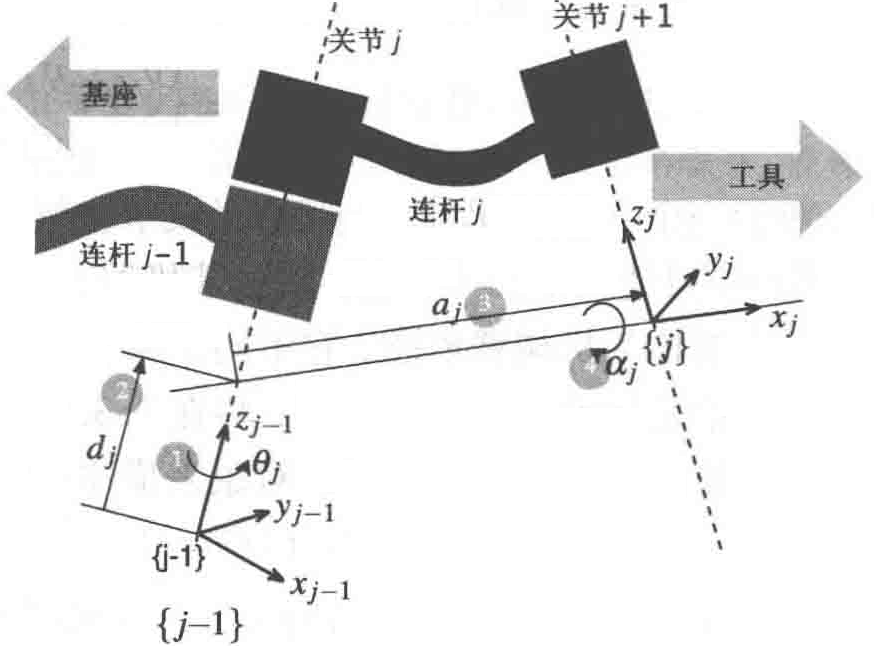如图所示。$\{j\}$ 坐标系固接于连杆 $j$ 的远端或末梢。关节 $j$ 的轴线即为该坐标系的 $z$ 轴。这些连杆和关节的参数即我们所熟知的 D-H 参数，总结于下表中。

参数
符号
物理含义
定义

关节角
$\theta_j$
$x_{j-1}$ 轴和 $x_j$ 轴之间关于 $z_{j-1}$ 轴的角度
转动关节变量

连杆偏移
$d_j$
沿着 $z_{j-1}$ 轴，$j-1$ 坐标系原点到 $x_j$ 轴的距离
移动关节变量

连杆长度
$a_j$
沿着 $x_j$ 轴，$z_{j-1}$ 轴和 $z_j$ 轴之间的距离；对于相交轴，它与 $\hat{z}_{j-1}\times\hat{z}_j$ 平行
常量

连杆扭转角
$\alpha_j$
$z_{j-1}$ 轴和 $z_j$ 轴之间关于 $x_{j}$ 轴的角度
常量

关节类型
$\sigma_j$
$\sigma=0$为转动副，$\sigma=1$为移动副
常量

按照约定，第一个关节，即关节 $1$，连接连杆 $0$ 和连杆 $1$。连杆 $0$ 为机器人的基座。通常来说第一个连杆有 $d_1=\alpha_1=0$，然而我们可以设定 $d_1>0$ 用来表示肩关节的高度超出基座。在一个制造系统中，基座通常是固定在环境中的，然而它有可能安装在一个移动的基座上，例如一架航天飞机、一个水下机器人或者一辆卡车。
最后一个关节，即关节 $N$，连接连杆 $N-1$ 和连杆 $N$。连杆 $N$ 是机器人的工具，它的相关参数 $d_N$ 和 $a_N$ 分别代表了工具的长度及其 $x$ 轴的偏移量。如图所示，这个工具通常指向 $z$ 轴。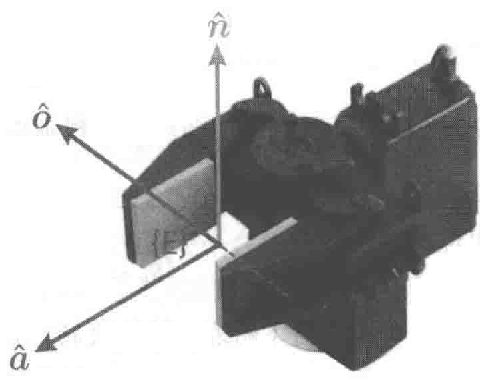从连杆坐标系 $\{j-1\}$ 到坐标系 $\{j\}$ 的变换被定义为基本旋转和平移，其形式如下：
$^{j-1}A_j(\theta_j,d_j,a_j,\alpha_j)=T_{Rz}(\theta_j)T_z(d_j)T_x(a_j)T_{Rx}(\alpha_j)$展开如下
$^{j-1}A_j=\left( \begin{matrix} \cos \theta _j& -\sin \theta _j\cos \alpha _j& \sin \theta _j\sin \alpha _j& a_j\cos \theta _j\\ \sin \theta _j& \cos \theta _j\cos \alpha _j& -\cos \theta _j\sin \alpha _j& a_j\sin \theta _j\\ 0& \sin \alpha _j& \cos \alpha _j& d_j\\ 0& 0& 0& 1\\ \end{matrix} \right)$
参数 $a_j$ 和 $\alpha_j$，始终是常量。对于转动关节 $\theta_j$ 是关节变量且 $d_j$ 是常量，然而对于移动关节 $d_j$ 是变量，$\theta_j$ 是常量，且 $\alpha_j=0$。在很多公式中，我们将使用如下的广义关节坐标：
$q_j=\left\{ \begin{array}{l} \theta _j,\quad\sigma_j=0转动关节\\[1em] d_j,\quad\sigma_j=1移动关节\\ \end{array} \right.$对于一个 $N$ 轴的机器人，其广义关节坐标为 $q\in\mathcal{C}$，其中 $\mathcal{C}\subset\mathbb{R}^N$ 被称为关节空间或者位形空间。一般情况下，一个全旋转关节机器人 $\mathcal{C}\subset\mathbb{S}^N$ 的关节坐标被称为关节角度。这种关节坐标同时也被称为机械臂姿态，它不同于属于笛卡儿姿态 $\xi\in SE(3)$ 的末端执行器的姿态。
我们使用机器人工具箱中的Link对象来描述一个机器人连杆，其创建如下：

>> L = Link([0, 0.1, 0.2, pi/2, 0])
L =
theta=q, d=0.1, a=0.2, alpha=1.571, offset=0 (R,stdDH)

其中，输入向量的元素按顺序分别是 $\theta_j$，$d_j$，$a_j$，$\alpha_j$。而可选的第 $5$ 个元素 $\sigma_j$ 则表明该关节是转动的 $\sigma_j=0$ 还是移动的 $\sigma_j=1$ ，如果未指定该值则默认为转动关节。
该Link对象的显示值不仅给出了其运动学参数，还表示了其他的一些状态，例如它是一个转动关节（标签“R”），以及使用了标准的 D-H 参数表达（标签“stdDH”）。

尽管赋给了 $\theta$ 一个值，但并没有显示出来——这个值在运动学参数系列中仅仅作为一个占位者。$\theta$ 将被关节变量 $q$ 所取代，因此它的值在Link对象中被忽略掉了。这个值将由Link对象进行管理。

当 $q=0.5$ 时，连杆变换矩阵如下：
>> L.A(0.5)
ans =
0.8776   -0.0000    0.4794    0.1755
0.4794    0.0000   -0.8776    0.0959
0    1.0000    0.0000    0.1000
0         0         0    1.0000

这是一个 $4×4$ 的齐次变换矩阵。我们可以对不同连杆参数的值进行读取或更改。例如：
>> L.RP
ans =
'R'

它表明这个连杆是一个转动型的。而
>> L.a
ans =
0.2000

它返回运动参数 $a$ 的值。最后，一个连杆还能包含一个偏移量：
>> L.offset = 0.5;
>> L.A(0)
ans =
0.8776   -0.0000    0.4794    0.1755
0.4794    0.0000   -0.8776    0.0959
0    1.0000    0.0000    0.1000
0         0         0    1.0000

该值将在计算连杆变换之前加到关节变量中。


展开全文• 看了一篇文章，觉得里面的DH参数标定法讲的很清晰，便使用Matlab复现了一下，效果还可以，特写此文记录。 2.原理 2.1.误差最佳值的最小二乘计算 有研究结果表明：几乎95％的机器人位置误差都是由机器人内部运动...
1.前言

看了一篇文章，觉得里面的DH参数标定法讲的很清晰，便使用Matlab复现了一下，效果还可以，特写此文记录。

2.原理

2.1.误差最佳值的最小二乘计算

有研究结果表明：几乎95％的机器人位置误差都是由机器人内部运动学的不准确描述引起的，所以对DH模型参数进行标定是很有必要的。

DH参数物理含义：选自《机器人学、机器视觉与控制-Matlab基础》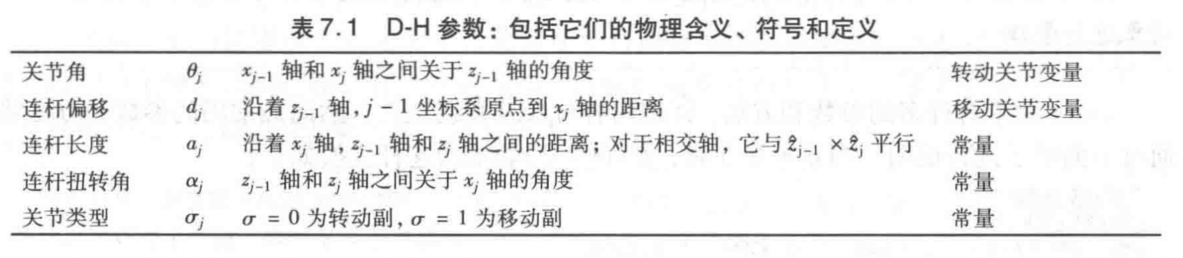当DH参数确定后，我们可以通过确定每根轴的角度值。在每根轴上建立相应的齐次方程而构建机械臂人的运动模型，就可以确定机械臂相对于机械臂的基座的末端位置和姿态。

通过将各个齐次矩阵联乘即可得到末端位姿和基坐标的转换矩阵，本文用表示：

后面的计算只会用到F的第4列

其中有末端位姿P：

为了简化求解运算，我们将用测量所得的连杆扭角值来代替理论的连杆扭角值α，同时认为通过机器人码盘读取的每根轴的转角值θ不存在误差，末端位姿可以简化为：

注意，我们在初次计算P的时候，DH参数采用的是厂家给的理想值！是需要进行标定的！

因为DH参数存在误差，所以真实的机械臂末端位姿为：

理想位姿和实际位姿的误差为：

*******当DH误差都比较小时，可以简化成相应的线性方程：（此处不是很懂）**********

因此对于一个末端位姿xyz，我们有三个方程成立，此三个方程成为一组位姿的方程，分别为x y z三个方向上的偏差：

对于我们六关节机器人，则有6个d和a参数，故一组方程为一个3x6的方程组，同时也是一组雅可比：

将其写为矩阵形式：

其中：

A为雅可比矩阵，每3行为一组，共有n组，其中n为我们所取的测试位姿个数，也就是说如果我们采样了n个位姿进行标定，则会产生3n*12维的A矩阵

B为n组位姿的误差矩阵，维数为3n*1：

δX也就是我们要求的标定参数：DH参数的误差，为一个12*1维的矩阵

***************只要我们样本n取得足够大，就可以利用最小二乘法求得δX的最佳值*****************

其中，δX为12*1维，inv(AT*A)为12*12维，AT为12*3n维，B为3n*1维，显然维数匹配

2.2.求到误差了干啥？

每次求到了误差δX，我们便将δX叠加到厂家给的DH参数上，获得新的DH参数，再重新求解误差，反复迭代，直到位姿的xyz误差足够小为止，此时的

δX+厂家DH参数=实际DH参数

3.Matlab代码实现

代码已上传到GitHub：我的GitHub

算法要点1

每次迭代时，都是将上一步求得的误差系数δX叠加到理论DH参数上，也就是厂家给的DH参数

    d1 = d1_idea + delta_x(1)
d2 = d2_idea + delta_x(2)
d3 = d3_idea + delta_x(3)
d4 = d4_idea + delta_x(4)
d5 = d5_idea + delta_x(5)
d6 = d6_idea + delta_x(6)
a1 = a1_idea + delta_x(7)
a2 = a2_idea + delta_x(8)
a3 = a3_idea + delta_x(9)
a4 = a4_idea + delta_x(10)
a5 = a5_idea + delta_x(11)
a6 = a6_idea + delta_x(12)

算法要点2

误差计算时是以理论xyz位姿减去实际xyz位姿，本程序以puma560机械臂为例子

    dP = p560.fkine(qn).t - F1;
B1 = dP;

其中
F = p560_real.fkine([q1, q2, q3, q4, q5, q6]).t   %F矩阵只要正运动学中xyz部分，不要角度部分

算法要点3

因为AT*A不是一个满秩的矩阵，所以不能使用inv函数求逆，要使用广义逆函数：pinv求逆

delta_x = pinv(Ao'*Ao)*Ao'*Bo;    %误差系数

算法要点4

使用Matlab符号函数，虽然速度很慢，但是最终还是得到了满意的结果

syms d1 d2 d3 d4 d5 d6;   %机械臂连杆偏移
syms a1 a2 a3 a4 a5 a6;     %机械臂连杆长度
syms q1 q2 q3 q4 q5 q6;    %转动角度

p560_real(1) = Link([0, d1, a1, 1.5708, 0]);
p560_real(2) = Link([0, d2, a2, 0, 0]);
p560_real(3) = Link([0, d3, a3, -1.5708, 0]);
p560_real(4) = Link([0, d4, a4, 1.5708, 0]);
p560_real(5) = Link([0, d5, a5, -1.5708, 0]);
p560_real(6) = Link([0, d6, a6, 0, 0]);
p560_real = SerialLink(p560_real, 'name', 'p560_real');    %建立真实的puma560模型，参数比理论测量大0.01
disp('建立完成')

F = p560_real.fkine([q1, q2, q3, q4, q5, q6]).t   %F矩阵只要正运动学中xyz部分，不要角度部分

A = [
diff(F, a1), diff(F, a2), diff(F, a3), diff(F, a4), diff(F, a5), diff(F, a6), diff(F, d1), diff(F, d2), diff(F, d3), diff(F, d4), diff(F, d5), diff(F, d6)
]    %建立误差线性矩阵

其中，diff(F, a1)是在对F求a1的偏导

完成对符号参数赋值后，再将符号函数转为数值函数：

A1 = double(subs(A));

注意要用double()转换一下，不然仍为sym数据类型，就会出现不计算sin函数的情况，之前我就遇到了计算出来的结果中含有sin(332/22)这种样子的数据，并没有计算sin值，这就是因为没有转为数值函数，仍为sym符号数据类型

4.程序效果

人为设置偏差：delta_x = [0.012; 0.021; 0.013; 0.01; 0.01; -0.01; 0.01; 0.01; 0.01; 0.012; -0.01; -0.01];

也就是说机械臂的理想DH参数要加上这些人为设置的偏差才是实际的DH，我们需要通过该程序拟合出最佳的误差系数

迭代10次的效果：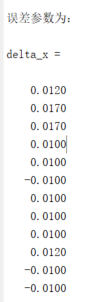可以发现，很接近了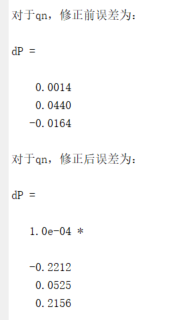且误差已经很小了，效果不错哦

参考文献

任永杰,邾继贵,杨学友, 等.利用激光跟踪仪对机器人进行标定的方法[J].机械工程学报,2007,43(9):195-200. DOI:10.3321/j.issn:0577-6686.2007.09.038.

展开全文• 5自由度机械手臂dh参数，运动学建模矩阵变换， .
• 机械手臂的一个重要特征参数就是DH参数。具体资料可查询：维基百科英文版：http://en.wikipedia.org/wiki/Denavit%E2%80%93Hartenberg_parameters由于被墙，现摘录如下： Denavit–Hartenberg parameters From ...
• 绕X轴旋转： 绕Y轴旋转： 绕Z轴旋转： ...ZX类变换：先绕着Zi-1（i-1坐标系）轴旋转和平移，然后绕Xi（i坐标系）轴旋转和平移 ...XZ类变换：先绕着Xi-1（i-1坐标系）轴旋转和平移，然后绕Zi（i坐标系）轴旋转和平移...
• 机械臂正运动学-DH参数-Python快速实现 文章目录机械臂正运动学-DH参数-Python快速实现前言：整体思路流程：学习资料核心概念：DH参数的理解。DH参数的定义：建立坐标系矩阵变换公式：最后直接上代码吧： 前言： ...机器人学 转换矩阵
• 6. 正运动学标准DH参数matlab代码： clear; clc; syms theta1 theta2 theta3 theta4 theta5 theta6; syms alpha1 alpha2 alpha3 alpha4 alpha5 alpha6; syms a1 a2 a3 a4 a5 a6; syms d1 d2 d3 d4 d5 d6; T1 ...
• 机械手臂建模软件实践D-H参数的求解 D-H参数的求解python anaconda
• dh参数 1. 标准的STD_DH dh建模 Trans zi-1（di）:沿着zi-1轴平移di；Rot zi-1（）:沿着zi-1轴旋转；Trans xi（ai）:沿着xi轴配平移ai ；Rot xi-1（）沿着xi-1轴旋转 2.改进型MOD_DH dh建模 3. ...matlab
• 今天，我们要把目光收回到机器人身上，看看我们怎样用一系列坐标系来描述一个链式机械臂，机器人学常说的DH参数（Denavit–Hartenberg parameters）又是什么。   相邻关节的坐标变换 在第一篇文章（《干货...
• 2019/5/30 新编辑：已经有两个小伙伴证明了：我这个程序在我的MATLAB2017b上运行没问题，但在2018版本上就会报错，我也不知道是什么原因！！！ 2019/4/25新编辑：改正了zhengyundongxue和niyundon...
• DH参数就是一个用四个参数表达两对关节连杆之间位置角度关系的机械臂数学模型和坐标系确定系统 。通过下文我们会看到，它通过 限制原点位置和X轴的方向 ，人为减少了两个自由度，因此它只需要用四个参数即可表达关节...
• 如下参考链接1的作者大大实现了UR5机械臂的正运动学和逆运动学的...参考链接：六轴UR机械臂正逆运动学求解_MATLAB代码（标准DH参数表） UR机械臂正逆运动学求解 一、参考链接说明 参考链接2的作者实现了UR5机械.Matlab
• schunkRobot 机器人运动matlab仿真 建立5关节schunk机械臂DH参数模型，进行matlab仿真 实现空间中8个目标点位置的运动
• 我们在求解机械臂的正运动学的时候，对于在写论文的过程，我们需要对最终的位姿矩阵进行相应的化简，比如把sin(q1) 写成 S1,cos(q1) 写成 C1等等之类，之前我在处理这些表达式的时候，都... 一般在DH参数中 theta 和...Matlab
• 1.在机械手臂中有两个重要参数。一个是编码器的值，另外一个是马达的电流值。根据这两个可以获得机械手臂的运动学，动力学的一些数据。...第一重要特征参数 是DH参数，另外一个就是每个轴的质心参数。
• 六自由度机械臂研究（2）- 机械臂坐标系建立， DH参数表建立 1. 机械臂坐标系建立 机械臂的坐标系建立是未来解析推导运动学算法的基础， 也是机械臂入学者的第一个坑。 坑的原因是网上资料无数， 但是几乎没有任何...
• ## 机械臂学习思路

千次阅读 2020-06-03 17:09:58
2020年初的时候，为了完成毕业设计的任务，开始尝试学习机器人...自由度的设置☞机械臂位姿的定义☞旋转与平移的概念☞齐次坐标与齐次变换的定义☞标准dh参数表和改进dh参数表的绘画与设计☞机械臂的运动学☞雅各比矩阵
• ## DH参数与运动学

千次阅读 2017-10-09 22:46:19
我们使用矩阵相乘表示机械臂末端中心点(Tool Central Point, TCP)，如 M60=T10T21T32T43T54T65 M_0^6= T_0^1 T_1^2 T_2^3 T_3^4 T_4^5 T_5^6 其中各个Ti+1iT_i^{i+1}应用DH参数表示齐次变换是可以直接求逆的! 还...
• 对于一个机械臂，这里已旋转副连接为例，描述需要用到两个参数，连杆转角和连杆长度。这连个都是机械臂本身结构决定的参数。用于描述机械臂两端连接轴的数学关系，转角和公垂线的距离 在用于描述两个机械臂之间的...人工智能
• FreeCAD中的机械臂模型是由.wrl和.csv文件两部分构成的，在...其中.wrl文件主要是用来定义机械臂的外形特征和几何连接关系，.csv文件是用来保存6轴机械臂DH参数和转角及速度约束。文档中具体介绍如何建立这两个文件。
• ## UR机械臂正逆运动学求解

万次阅读 多人点赞 2018-07-21 16:14:10
最近有个任务：求解UR机械臂正逆运动学，在网上参考了... 以UR5机械臂结构和尺寸参数为例进行正逆运动学求解，下图分别是UR5结构图和标准DH参数： 1. 正运动学求解 正运动学是已知关节六个角度求变换矩...